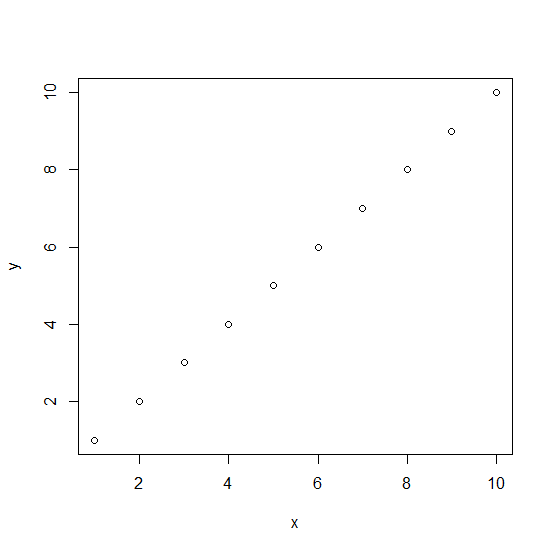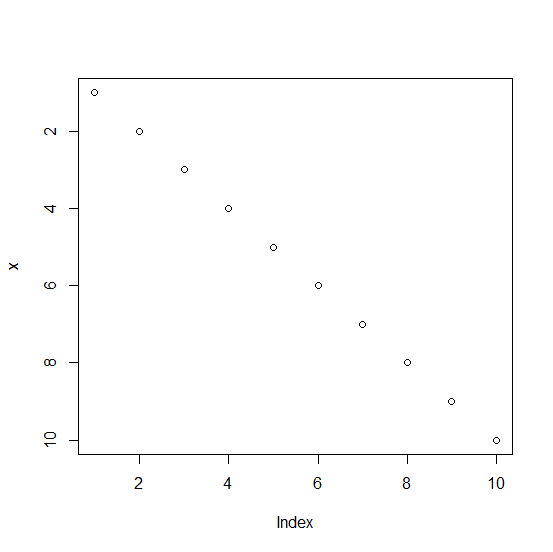# How to create a plot with reversed Y-axis labels in base R?

To create a plot with reversed Y-axis we need to use the rev function for the Y-axis labels with ylim but we would also need to define the range for the y-axis values, otherwise, R will throw an error. For example, if we have two vectors named as x and y then the plot with reversed Y-axis labels can be created by using plot(x,ylim=rev(range(y))).

## Example

Live Demo

x<-1:10
y<-1:10
plot(x,y)

## OutputCreating the plot with reversed Y-axis labels −

## Example

plot(x,ylim=rev(range(y)))

## Output